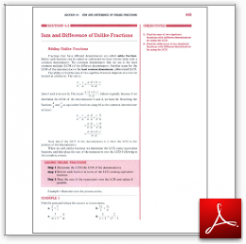Algebra Math Book - Introductory Algebra Thank you for your continual support.

 The power of mathematics rests in the logic of thinking.ID: Sec6-6
Description: Adding and Subtracting Unlike Fractions
Price: 1.99

# Algebra Math book - Introductory Algebra - Chapter 6 - Section 6 - Sum and Difference of Unlike Fractions

## Section 6.6 - Objectives

8.  Find the sum of two algebraic fractions with different denominators using the LCD.

9.  Find the difference of two algebraic fractions with different denominators by using the LCD.

Navigate to
Previous Section or Next Section
or Chapter 6 Details or Other Chapters

This section of my algebra math book, Introductory Algebra, also includes in the download:

• Cover Sheet